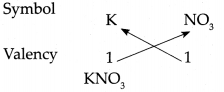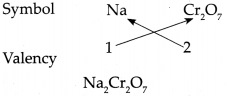Balbharti Maharashtra State Board Class 9 Science Solutions Chapter 4 Measurement of Matter Notes, Textbook Exercise Important Questions and Answers.

## Maharashtra State Board Class 9 Science Solutions Chapter 4 Measurement of Matter

Class 9 Science Chapter 4 Measurement of Matter Textbook Questions and Answers

1. Give examples.

Na+– Sodium ion, K+ – Potassium ion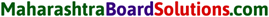Na+ – Sodium ion, K+ – Potassium ion, Ag+ – Silver ion

$$\mathrm{SO}_{4}^{2-}, \mathrm{NH}_{4}^{+}$$

d. Metals with variable valency
(a) Iron (Ferrum)
(i) Fe2+ – Ferrous [Iron – II]
(ii) Fe3+ – Ferric [Iron – III]

(b) Copper (Cuprum)
(i) Cu+ – Cuprous [Copper -1]
(ii) Cu2+ – Cupric [Copper – II]

(c) Mercury (Hydragyrum)
(i) Hg+ – Mercurous [Mercury -1]
(ii) Hg2+ – Mercuric [Mercury – II]

O2- – Oxide, S2- – Sulphide, $$\mathrm{CO}_{3}^{2-}$$ – Carbonate

Al3+ – Aluminium, Cr3+ – Chromium, Fe3+ – Ferric.

2. Write symbols of the following elements and the radicals obtained from them, and indicate the charge on the radicals.
Mercury, potassium, nitrogen, copper, sulphur, carbon, chlorine, oxygen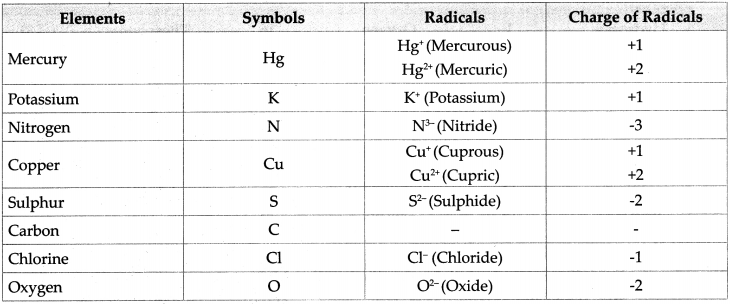3. Write the steps in deducing the chemical formulae of the following compounds.
Sodium sulphate, potassium nitrate, ferric phosphate, calcium oxide, aluminium hydroxide
In order to write the chemical formulae of compounds, it is necessary to know the symbols and valency of various radicals.

1. Sodium Sulphate:
Step – 1 : To write the symbols of the radicals (Basic radicals on the left and acidic radicals on the right)
$$\mathrm{Na} \quad \mathrm{SO}_{4}$$
Step – 2 : To write the valency below the respective radical.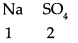Step – 3: To cross-multiply as shown by arrows the number of radicals.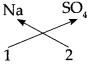Step – 4 : To write down the chemical formula of the compound.
Na2 SO4
(Sodium sulphate)

2. Potassium Nitrate:
Step -1 : To write the symbols of the radicals (Basic radicals on the left and acidic radicals on the right)
K NO3
Step – 2 : To write the valency below the respective radical.
$$\begin{array}{cc} \mathrm{K} & \mathrm{NO}_{3} \\ 1 & 1 \end{array}$$
Step – 3: To cross-multiply as shown by arrows the number of radicals.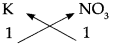Step – 4 : To write down the chemical formula of the compound.
KNO3
(Potassium nitrate)

3. Ferric phosphate:
Step -1 : To write the symbols of the radicals (Basic radicals on the left and acidic radicals on the right)
Fe PO4
Step – 2 : To write the valency below the respective radical.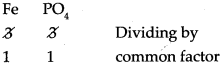Step – 3: To cross-multiply as shown by arrows the number of radicals.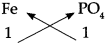Step – 4 : To write down the chemical formula of the compound.
FePO4
(Ferric phosphate)4. Calcium oxide:
Step – 1 : To write the symbols of the radicals (Basic radical on the left and acidic radicals on the right)
Ca O
Step – 2 : To write the valency below the respective radical.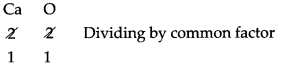Step – 3: To cross-multiply as shown by arrows the number of radicals.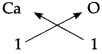Step – 4 : To write down the chemical formula of the compound.
CaO
(Calcium oxide)

5. Aluminium hydroxide:
Step – 1 : To write the symbols of the radical (Basic radical on the left and acidic radical on the right)
Al OH
Step – 2 : To write the valency below the respective radical.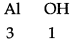Step – 3: To cross-multiply as shown by arrows the number of radicals.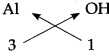Step – 4 : To write down the chemical formula of the compound.
Al(OH)3
(Aluminium hydroxide)

6. Calcium carbonate:
Step – 1 : To write the symbols of the radical (Basic radical on the left and acidic radicals on the right)
Ca CO3
Step – 2 : To write the valency below the respective radical.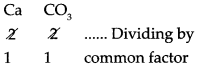Step – 3: To cross-multiply as shown by arrows the number of radicals.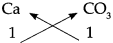Step – 4 : To write down the chemical formula of the compound.
CaCO3
(Calcium Carbonate)

7. Sodium dichromate:
Step – 1 : To write the symbols of the radicals (Basic radical on the left and acidic radical on the right)
Na Cr2O7
Step – 2 : To write the valency below the respective radical.
$$\begin{array}{cc} \mathrm{Na} & \mathrm{Cr}_{2} \mathrm{O}_{7} \\ 1 & 2 \end{array}$$
Step – 3: To cross-multiply as shown by arrows the number of radicals.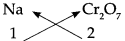Step – 4 : To write down the chemical formula of the compound.
Na2Cr2O7
(Sodium dichromate)a. Explain how the element sodium is monovalent.

1. The number of protons or electrons (atomic number) in Sodium (Na) atom is 11. Therefore the electronic configuration of sodium atom is (2, 8,1).
2. In chemical reaction, sodium atom has the capacity to give away le_ from its outermost orbit to form Na+ ion with stable electronic configuration (2, 8).
3. As sodium atom gives away le- and a cation of sodium is formed, hence the valency of sodium is 1 and therefore, the element sodium is monovalent.

b. M is a bivalent metal. Write down the steps to find the chemical formulae of its compounds formed with the radicals, sulphate and phosphate.
M is a bivalent metal. Following are the steps to find the chemical formulae of its compounds formed with the radicals, sulphate and phosphate:

(i) Compound of metal ‘M’ with radical sulphate
Step – 1: To write the symbols of the radicals (Basic radicals on the left and acidic radicals on the right)
M SO4
Step – 2: To write the valency below the respective radical.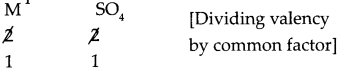Step – 3: To cross multiply as shown by arrows the number of radicals.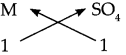Step – 4: To write down the chemical formula of the compound.
M SO4

(ii) Compound of metal ‘M’ with radical phosphate.
Step – 1: To write the symbols of the radicals (Basic radicals on the left and acidic radicals on the right)
M PO4
Step – 2: To write the valency below the respective radical.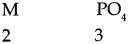Step – 3: To cross multiply as shown by arrows the number of radicals.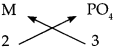Step – 4: To write down the chemical formula of the compound.
M3 (PO4)2c. Explain the need for a reference atom for atomic mass. Give some information about two reference atoms.

• The mass of an atom is concentrated in its nucleus and it is due to the protons (p) and neutrons (n) in it.
• Since an atom is very very tiny, it was not possible to measure atomic mass accurately. Therefore, the concept of relative mass of an atom was formed.
• To express relative mass of an atom, reference of atom is considered. The two reference atoms were as follows:

(a) Hydrogen (H) atom: The hydrogen atom is the lightest. The relative mass of a hydrogen atom is 1 which has only 1 proton in its nucleus. On this scale, the relative atomic mass of many elements comes out to be fractional. Therefore, carbon was selected as a reference atom.

(b) Carbon (C) atom: The carbon atom is selected as reference atom. In this scale, the relative mass of a carbon atom is accepted as 12.

• The relative atomic mass of 1 hydrogen (H) atom compared to the carbon (C) atom becomes

d. What is meant by Unified Atomic Mass.

• During earlier time, relative mass of an atom was considered for measuring the mass of an atom directly. But since the founding of unified mass, relative mass is not accepted henceforth.
• Unified atomic mass is the unit of atomic mass called as Dalton.
• Its symbol is ‘u’. lu = 1.66053904 x 10-27 kg.

e. Explain with examples what is meant by a ‘mole’ of a substance.

• A mole is that quantity of a substance whose mass in grams is equal in magnitude to the molecular mass of that substance in Daltons.
• For example: Atomic mass of oxygen atom (O) is 16u. Thus, the molecular mass of oxygen molecule (O2) is 16 x 2 = 32u. Therefore, 32 g of oxygen is 1 mole of oxygen.

5. Write the names of the following compounds and deduce their molecular masses.
Na2SO4, K2CO3, CO2, MgCl2, NaOH, AlPO4, NaHCO3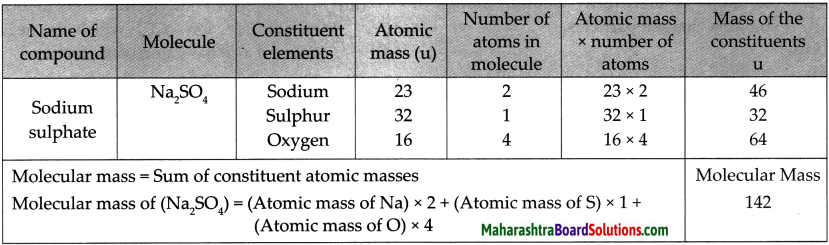6. Two samples ‘m’ and ‘n’ of slaked lime were obtained from two different reactions. The details about their composition are as follows:
‘sample m’ mass : 7g
Mass of constituent oxygen : 2g
Mass of constituent calcium : 5g
‘sample n’ mass : 1.4g
Mass of constituent oxygen : 0.4g
Mass of constituent calcium : 1.0g

Which law of chemical combination does this prove? Explain.
(i) The expected proportion by weight of the constituent elements of quick lime that is calcium oxide would be from its known molecular formula CaO. The atomic mass of Ca and O are 40 and 16 respectively. This means, the proportion by weight of the constituent elements Ca and O in the compound CaO is 40 :16 which is 5 : 2.

(ii) Now, for the given sample’m’ of CaO = 5 g
mass of given sample = 7 g
mass of constituent Ca in sample’m’ = 5 g
mass of constituent O in sample’m’ = 2 g

(iii) This means that 7 g of calcium oxide contairis 5 g of calcium (Ca) and 2 g of oxygen (O); apd the proportion by weight of calcium and oxygen in it is 5 : 2.

(iv) Now, for the given sample ‘n’ of CaO mass of given sample CaO = 1.4 g
Mass of constituent Ca in sample ‘n’ = 1.0 g
Mass of constituent O in sample ‘n’ = 0.4 g
This means that 1.4g of calcium oxide contains 1.0 g of calcium (Ca) and 0.4 g of oxygen (O); and the proportion by weight of calcium and oxygen in it is 5 : 2.

(v) Above samples’m’ and ‘n’ of calcium oxide (CaO) shows that the proportion by weight of the constituent elements in different samples of a compound is always constant that is the proportion by weight of calcium (Ca) and oxygen (O) in different samples of calcium oxide (CaO) is constant.

(vi) The experimental value of proportion by weight of the constituent elements matched with the expected proportion calculated by molecular mass. This proves and verifies the law of constant proportion.

The law states that ‘The proportion by weight of the constituent elements in the various samples of a compound is fixed’.7. Deduce the number of molecules of the following compounds in the given quantities.
32g oxygen, 90g water, 8.8g carbon dioxide, 7.1g chlorine.

Class 9 Science Chapter 4 Measurement Of Matter Notes Question 1.
32g oxygen
Given : Mass of oxygen (O2) m = 32g
To find : Number of molecules in 32g of oxygen.
Solution : Atomic mass of oxygen (O) = 16
∴ Molecular mass of oxygen (O2) M = 16 x 2 = 32
According to the formula, Number of moles in the given O2 (n)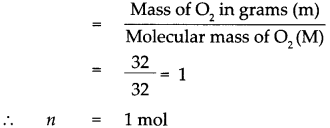1 mol of O2 contains 6.022 x 1023 molecules that is 32 g of 02 contains 6.022 * 1023 molecules of O2.
32g of oxygen contains 6.022 x 1023 molecules of oxygen.

Class 9 Science Chapter 4 Measurement Of Matter Answers Question 2.
90g water
Given : Mass of water (H2O) m = 90g.
To find : Number of molecules in 90g of water.
Solution : Molecular mass of (H2O) M = (Atomic mass of H) x 2 + (Atomic mass of O) x 1
∴ Molecular mass of (H2O) M = 1 x 2 +16
∴ Molecular mass of (H2O) M = 18
According to the formula,
Number of moles in the given H2O (n)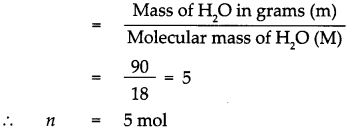1 mol of H2O contains 6.022 x 1023 molecules.
5 mol of H2O contains 5 x 6.022 x 1023 molecules. = 30.11 x 1023 molecules, that is 90g of H2O contains 30.11 x 1023 molecules of H20.
90g of water contains 30.11 x 1023 molecules of water.4 Measurement Of Matter Exercise Question 3.
8.8g carbon dioxide
Given : Mass of Carbon dioxide (CO2)m = 8.8g.
To find : Number of molecules in 8.8g of carbon dioxide.
Solution : Molecular mass of (CO2)M = (Atomic mass of C) x 1 + (Atomic mass of O) x 2
∴ Molecular mass of (CO2)M = 12 x 1 + 16 x 2 = 12 + 32
Molecular mass of (CO2)M = 44
According to the formula, Number of moles in the given CO2 (n)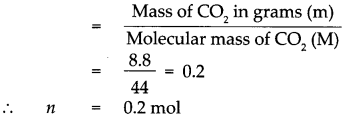∴ 1 mol of CO2 contains 6.022 x 1023 molecules.
∴ 0.2 mol of CO2 contains 0.2 x 6.022 x 1023 molecules.
= 1.2044 x 1023 molecules,
that is 8.8g of CO2 contains 1.2044 x 1023 molecules of CO2.
8.8g of CO2 contains 1.2044 x 1023 molecules of CO2.

Class 9 Science Solutions Maharashtra Board Question 4.
7.1g chlorine
Given : Mass of Chlorine (Cl2)m = 7.1g.
To find : Number of molecules in 7.1g of chlorine.
Solution : Atomic mass of (Cl) = 35.5
∴ Molecular mass of chlorine (Cl2)M = 35.5 x 2 = 71
According to the formula, Number of moles in the given Cl2 (n)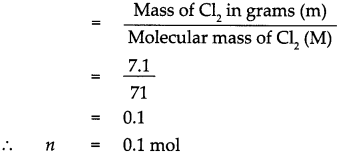∴ 1 mol of Cl2 contains 6.022 x 1023 molecules.
∴ 0.1 mol of Cl2 contains 0.1 x 6.022 x 1023 molecules.
= 0.6022 x 1023 molecules,
that is 7.1g of Cl2 contains 0.6022 x 1023 molecules of Cl2.
7.1g of Cl2 contains 0.6022 x 1023 molecules of chlorine.

8. If 0.2 mol of the following substances are required how many grams of those substances should be taken? Sodium chloride, magnesium oxide, calcium carbonate
Given : Number of moles of sodium chloride (NaCl) n = 0.2 mol
To find : Mass in grams of 0.2 mol of NaCl
Solution:
Molecular mass of (NaCl)M = (Atomic mass of Na) x 1 + (Atomic mass of Cl) x 1
= 23 x 1 + 35.5 x 1
= 23 + 35.5
Molecular mass of (NaCl)M = 58.5
According to the formula,
Number of moles in the given NaCl (n)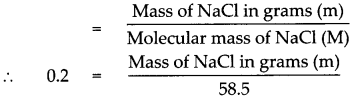Mass of NaCl in grams (m) = 0.2 x 58.5
Mass of NaCl in grams (m) = 11.7 g
Mass of 0.2 mole of NaCl is 11.7gClass 9 Science Chapter 3 Current Electricity Intext Questions and Answers

Maharashtra State Board Class 9 Science Solutions Question 1.
What is the type of chemical bond in NaCl and MgCl2?

• The type of chemical bond in NaCl and MgCl2 is ionic bond.

9th Class Science Chapter 4 Measurement Of Matter Question 2.
Determine the valencies of H, Cl, O and Na from the molecular formulae H2, HC1, H2O and NaCl.
(i) In the molecular formula HCl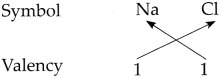∴ The valency of H is 1 and Cl is 1.

(ii) In the molecular formula H2O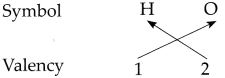∴ The valency of H is 1 and O is 2.

(iii) In the molecular formula NaCl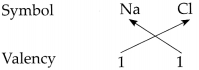∴ The valency of Na is 1 and Cl is 1.
∴ From all the above, the valencies of the given elements are as follows : H = 1, Cl = 1, O = 2 and Na = l.

Measurement Of Matter Class 9 Exercise Answers Question 3.
How is an element indicated in Chemistry?
In chemistry an element is indicated by its symbol.

Question 4.
Write down the symbols of the elements you know.
Symbols of some elements are

• Hydrogen – H
• Helium – He
• Boron – B
• Carbon – C
• Aluminium – A1

Question 5.
Write down the symbols for the following elements.
Antimony, Iron, Gold, Silver, Mercury, Lead, Sodium
The symbols of given elements are as follows:

• Antimony – Sb
• Iron – Fe
• Gold – Au
• Silver – Ag
• Mercury – Hg
• Sodium – NaFollowing are atomic masses of a few elements in Daltons and the molecular formulae of some compounds. Deduce the molecular masses of those compounds:

Atomic masses – H(l), 0(16), N(14), C(12), K(39), S(32) Ca(40), Na(23), Cl(35.5), Mg(24), Al(27)

Question 1.
Molecular formula – NaCl
Molecular mass of NaCl (M)
= (Atomic mass of Na) x 1 + (Atomic mass of Cl) x 1
= (23 x 1) + (35.5 x 1)
= 23 + 35.5
= 58.5
∴ Molecular mass of NaCl (M) = 58.5

Question 2.
Molecular formula – MgCl2
Molecular mass of MgCl2 (M)
= (Atomic mass of Mg) x 1 + (Atomic mass of Cl) x 2
= (24 x 1) + (35.5 x 2)
= 24 + 71
= 95
∴ Molecular mass of MgCl2 (M) = 95?

Question 3.
Molecular formula – KNO3
Molecular mass of KNO3 (M)
= (Atomic mass of K) x 1 + (Atomic mass of N) x 1 + (Atomic mass of O) x 3
= (39 x 1) + (14 x 1) + (16 x 3)
= 39 + 14 + 48
= 101
Molecular mass of KNO3 (M) = 101

Question 4.
Molecular formula – H2O2
Molecular mass of H2O2 (M)
= (Atomic mass of H) x 2 + (Atomic mass of O) x 2
= (1 x 2) + (16 x 2)
= 2 + 32
= 34
∴ Molecular mass of H2O2 (M) = 34.Question 5.
Molecular formula – A1C13
Molecular mass of A1C13 (M)
= (Atomic mass of Al) x 1 + (Atomic mass of Cl) x 3
= (27 x 1) + (35.5 x 3)
= 27 + 106.5
= 133.5
∴ Molecular mass of A1C13 (M) = 133.5

Question 6.
Molecular formula – Ca(OH)2
Molecular mass of Ca(OH)2 (M)
= (Atomic mass of Ca) x 1 + (Atomic mass of O + Atomic Mass of H) x 2
= (40 x 1) + (16 + 1) x 2
= 40 + (17 x 2)
= 40 + 34
= 74
∴ Molecular mass of Ca(OH)2 (M)
= 74

Question 7.
Molecular formula – MgO
Molecular mass of MgO (M)
= (Atomic mass of Mg) x 1 + (Atomic mass of 0)xl
= (24 x 1) + (16 x 1)
= 24 + 16
= 40
Molecular mass of MgO (M) = 40Question 8.
Molecular formula – H2S04
Molecular mass of H2S04 (M)
= (Atomic mass of H) x 2 + (Atomic mass of S) x 1 + (Atomic mass of O) x 4
= (1 x 2) + (32xl) + (16×4)
= 2 + 32 + 64
= 98
Molecular mass of H2S04 (M) = 98

Question 9.
Molecular formula – HN03
Molecular mass of HN03 (M)
= (Atomic mass of H) x 1 + (Atomic mass of N) x 1 + (Atomic mass of O) x 3
= (lxl)+ (14xl)+ (16×3)
= 1 + 14 + 48
= 63
Molecular mass of HNOs (M) = 63

Question 10.
Molecular formula – NaOH
Molecular mass of NaOH (M)
= (Atomic mass of Na) x 1 + (Atomic mass of O) x 1 + (Atomic mass of H) x 1
= (23 x 1) + (16 x 1) + (l x l)
= 23 + 16 + 1
= 40
Molecular mass of NaOH (M) = 40

Question 11.
How many molecules of water are there in 36 g water?
Given : Mass of water (H2O) m = 36g
To find : Number of molecules in 36g of water
Solution :
Molecular mass of (H2O) M = (Atomic mass of H) x 2 + (Atomic mass of O) x 1 Molecular mass of (H2O) M
= (1 x 2) + 16 x 1
Molecular mass of (H2O) M = 18
According to the formula,
Number of moles in the given H2O (n)1 mol of H2O contains 6.022 x 1023 molecules.
∴ 2 mol of H2O contains 2 x 6.022 x 1023 molecules.
= 12.044 x 1023 molecules, that is 36g of H2O contains 12.044 x 1023 molecules of H2O.
36 g of water contains 12.044 x 1023 molecules of water.Question 12.
How many molecules of H2S04 are there in a 49 g sample?
Given : Mass of Sulphuric acid (H2SO4) m = 49g
To find : Number of molecules in 49g of H2SO4
Solution:
Molecular mass of (H2SO4) M = (Atomic mass of H) x 2 + (Atomic mass of S) x 1 + (Atomic mass of O) x 4
Molecular mass of (H2SO4)M = (1 x 2) + (32 x 1) + (16 x 4)
= 2 + 32 + 64
= 98.
According to the formula,
Number of moles in the given H2SO4 (n)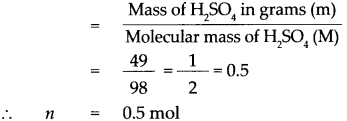∴ 1 mol of H2SO4 contains 6.022 x 1023 molecules.
∴ 0.5 mol of H2SO4 contains 0.5 x 6.022 x 1023 molecules.
= 3.011 x 1023 molecules,
that is 49g of H2SO4 contains 3.011 x 1023 molecules of H2SO4.
49 g of Sulphuric acid contains 3.011 x 1023 molecules of H2SO4.

Question 13.
Fill the following tables.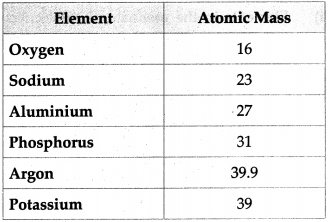Question 14.
Complete the following chart.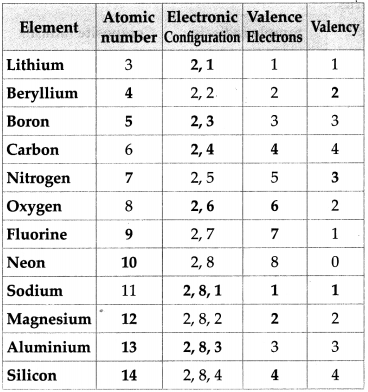Question 15.
The relative atomic masses of some elements in the chart below are given. You have to find the relative atomic masses of the others.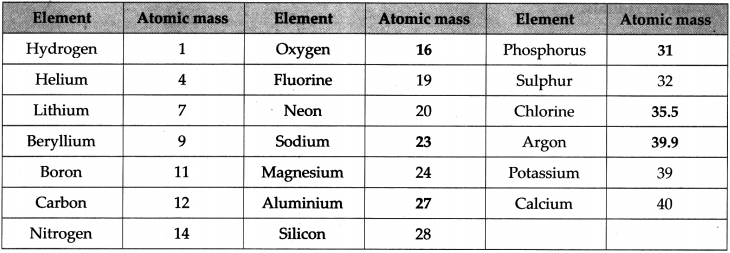Question 16.
$$\begin{array}{l} \mathrm{Ag}^{+}, \mathrm{Mg}^{2+}, \mathrm{Cl}^{-}, \mathrm{SO}_{4}^{2-}, \mathrm{Fe}^{2+}, \mathrm{ClO}_{3}^{-}, \mathrm{NH}_{4}^{+}, \mathrm{Br}^{-} \\ \mathrm{NO}_{3}^{-}, \mathrm{Na}^{+}, \mathrm{Cu}^{+} \end{array}$$

 Simple radicals Composite radicals Ag+ $$\mathrm{SO}_{4}^{2-}$$ Mg2+ $$\mathrm{ClO}_{3}^{-}$$ Cl $$\mathrm{NH}_{4}^{+}$$ Fe2+ $$\mathrm{NO}_{3}^{-}$$ Br– Na+ Cu+

Question 17.
Which are the basic radicals and which are the acidic radicals among the following?
$$\begin{array}{l} \mathrm{Ag}^{+}, \mathrm{Cu}^{2+}, \mathrm{Cl}^{+}, \mathrm{I}, \mathrm{SO}_{4}^{2-}, \mathrm{Fe}^{3+}, \mathrm{Ca}^{2+}, \mathrm{NO}_{3} ; \mathrm{S}^{2}, \mathrm{NH}_{4}^{+} \\ \mathrm{K}^{+}, \mathrm{MnO}_{4}, \mathrm{Na}^{+} \end{array}$$

 Basic Radical Acidic Radical (i)Ag+ (i) Cl– (ii) Cu2+ (ii) I– (iii) Fe3+ $$\text { (iii) } \mathrm{SO}_{4}^{2-}$$ (iv) Ca2+ $$\text { (iv) } \mathrm{NO}_{3}^{-}$$ Wnh; $$\text { (v) } \mathrm{S}^{2-}$$ (vi) K+ $$\text { (vi) } \mathrm{MnO}_{4}^{-}$$ (vii) Na+Give examples:

Question 1.
Make a list of elements in the monoatomic and in the diatomic molecular state. (Make a list and discuss;

• Elements in the monoatomic molecular state are: Helium (He), Neon (Ne), Argon (Ar), Sodium (Na), Copper (Cu),
• Elements in the diatomic molecular state are:
Oxygen (O2), Nitrogen (N2), Hydrogen (H2), Chlorine (Cl2), Fluorine (F2).

Problem-based questions

Question 1.
Is it possible to weigh one molecule using a weighing balance?
No, it is not possible to weigh one molecule using a weighing balance.

Question 2.
Will the number of molecules be the same in equal weights of different substances?
No, the number of molecules will not be the same in equal weights of different substances.

Question 3.
If we want equal number of molecules of different substances, will it work to take equal weights of those substances.
No, if we want equal number of molecules of different substances, it will not work to take equal weights of those substances.

Question 1.
What is the Dalton’s atomic theory?
Dalton’s Atomic theory-

• All matter is made of atoms. Atoms are indivisible and indestructible.
• All atoms of a given element are identical in mass and properties.
• Compounds are formed by a combination of two or more different kinds of atoms.
• A chemical reaction is a rearrangement of atoms.

Question 2.
How are compounds formed?
Compounds are formed by a chemical combination of two or more different kinds of atoms.Question 3.
What are the molecular formulae of salt, slaked lime, water, lime, limestone?
The molecular formulae for
Salt – Sodium chloride – NaCl
Slaked lime – Calcium hydroxide Ca(OH)2
Water – H2O
Lime – Calcium oxide – CaO
Lime stone – Calcium carbonate – CaCO3

Question 4.
From which experiments was it discovered that atoms have an internal structure? When?

• In 1911, Earnest Rutherford conducted a well known experiment called as ‘Gold foil experiment’.
• From this experiment it was discovered that atoms have internal structure.

Question 5.
What are the two parts of an atom? What are they made up of?
The two parts of atoms are nucleus and extra nuclear part. Nucleus is made up of positively charged protons and electrically neutral neutrons and the extra nuclear part is made up of negatively charged electrons revolving around the nucleus in different orbits.

Open-ended questions

Q.3. 2. Answer the following questions:

Question 1.
How will the compounds, MgCl2 and CaO be formed from their elements?
(1) Magnesium Chloride (MgCl2)
Magnesium atom (Mg): Electronic configuration
$$(2,8,2) \stackrel{-2 e^{-}}{\longrightarrow}$$ Magnesium ion Mg2+ (2,8).
Chlorine atom (Cl). Electronic configuration $$(2,8,7) \stackrel{+1 e^{-}}{\longrightarrow}$$ Chloride ion Ch (2,8,8).
∴ Mg2+ + 2CT → MgCl2 (Magnesium Chloride)

• A Magnesium atom gives away 2e and a cation of Magnesium (Mg2+) is formed, hence, the valency of magnesium is two.
• Two chlorine atoms takes le each and forms two anions of chlorine (2Cl) (chloride), and thus, the valency of chlorine is one.
• After the give and take of electrons is over, the electronic configuration of all the resulting ions has a complete octet.
• Due to the attraction between the unit but opposite charges on all the ions, one chemical bond known as ionic bond is formed between Mg2+ and 2C1 each and the compound MgCl2 is formed.

(2) Calcium Oxide (CaO)
Calcium atom (Ca): Electronic configuration
$$(2,8,8,2) \stackrel{-2 e^{-}}{\longrightarrow}$$ Calcium ion Ca2+ (2,8,8).
Oxygen atom (O). Electronic configuration (2,6)
$$\stackrel{+2 e^{-}}{\longrightarrow}$$ Oxygen ion O2- (2,8).
∴ Ca2+ + O2- → CaO

• A calcium atom gives away 2er and a cation of calcium (Ca2+) is formed, hence, the valency of calcium is two.
• An oxygen atom takes 2e and forms anions of oxygen (O2-) (oxide), and thus, the valency of oxygen is two.
• After the give and take of electrons is over, the electronic configuration of both the resulting ions has a complete octet.
• Due to the attraction between the unit but opposite charges on the two ions, one chemical bond known as ionic bond is formed between Ca2+ and O2- and the compound CaO is formed.Question 2.

• Take 56 g calcium oxide in a large conical flask and put 18 g water in it.
• Observe what happens.
• Measure the mass of the substance formed.
• What similarity do you find? Write your inference.

(i) When 18 g of water is added to 56 g of calcium oxide, calcium oxide combines with water to form calcium hydroxide Ca(OH)2

(ii) The mass of calcium hydroxide formed is 74 g.?

(iii) In this activity the total mass of reactants, Calcium oxide + Water = 56 g +18 g = 74 g.
It is equal to the mass of the product formed. Ca(OH)2 = 74g.

This activity verifies the Law of Conservation of Matter, i.e., in a chemical reaction, the total weight of the reactants is same as the total weight of the products formed due to the chemical reactions.

Question 3.

• Take a solution of calcium chloride in a conical flask and a solution of sodium sulphate in a test tube.
• Tie a thread to the test tube and insert it in the conical flask.
• Seal the conical flask with an airtight rubber cork.
• Weigh the conical flask using a balance.
• Now tilt the conical flask so that the solution in the test tube gets poured in the conical flask.
• Now weigh the conical flask again.

• In this activity, a white precipitate of CaS04 in NaOl is seen in the conical flask after the reaction.
• There is no change in the weight of the flask before and after the reaction.
• This activity verifies the Law of Conservation of Matter i.e., in a chemical reaction, the total weight of the reactants is same as the total weight of the products formed due to the chemical reactions.

Question 4.
Using the chart of ions/radicals and the cross-multiplication method, write the chemical formulae of the following compounds : Calcium carbonate, Sodium bicarbonate, Silver chloride, Calcium hydroxide, Magnesium oxide, Ammonium phosphate, Cuprous bromide, Copper sulphate, Potassium nitrate, Sodium dichromate.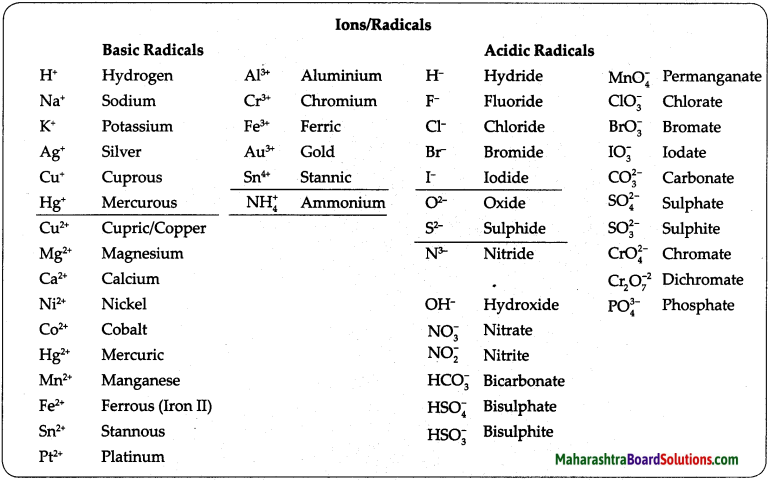Calcium carbonate – CaCO3 Sodium bicarbonate – NaHCO3 Silver chloride – AgCl, Calcium hydroxide – Ca(OH)2, Magnesium oxide – MgO, Ammonium phosphate – (NH4)3PO4, Cuprous bromide – CuBr, Copper sulphate – CuSO4, Potassium nitrate – KNO3, Sodium dichromate – Na2Cr2O7.Class 9 Science Chapter 3 Current Electricity Additional Important Questions and Answers

(A) Select the correct option:

Question 1.
The proportion by weight of hydrogen and oxygen in water is ……………………….. .
(a) 8 : 1
(b) 2 : 1
(c) 1 : 2
(d) 1 : 8
(d) 1: 8

Question 2.
The proportion by weight of carbon and oxygen in carbon dioxide is ……………………….. .
(a) 8 : 3
(b) 3 : 8
(c) 3 : 2
(d) 2 : 3
(b) 3 : 8

Question 3.
A nucleus of an atom is made up of positively charged ………………………… and electrically neutral ……………………….. .
(a) protons; neutrons
(b) electrons; neutrons
(c) neutrons; protons
(d) neutrons; electrons
(a) protons; neutrons

Question 4.
The size of an atom is determined by its ……………………….. .

Question 5.
Atomic radius is expressed in ……………………….. .
(a) milimetres
(b) centimetres
(c) nanometres
(d) picometres
(c) nanomet resQuestion 6.
The atomic size depends on the number of ………………………… in the atom.
(a) protons
(b) nucleus
(c) neutrons
(d) electron orbits
(d) electron orbits

Question 7.
The mass of an atom is concentrated in its ……………………….. .
(a) protons
(b) nucleus
(c) neutrons
(d) electrons
(b) nucleus

Question 8.
The total number of protons and neutrons in the atomic nucleus is called the ……………………….. .
(a) atomic number
(b) electronic configuration
(c) atomic mass number
(d) valency
(c) atomic mass number

Question 9.
A ………………………… is that quantity of a substance whose mass in grams is equal in magnitude to the molecular mass of that substance in Daltons.
(a) mole
(b) dalton
(c) dozen
(d) gross
(a) Mole

Question 10.
Avogadro’s number is denoted by the symbol ……………………….. .
(a) NG
(b) Nv
(c) NA
(d) ND
(c) NAQuestion 11.
A mole of any substance stands for ………………………… molecules.
(a) 60.22 x 1023
(b) 6.022 x 1022
(c) 6.022 x 1023
(d) 60.22 x 1022
(a) 60.22 x 1023

Question 12.
The capacity of an element to combine is called its ……………………….. .
(a) valency
(b) electronic configuration
(c) atomic number
(d) volence electrons
(a) valency

Question 13.
Electronic configuration of sodium atom is ……………………….. .
(a) (2, 8, 3)
(b) (2, 8, 7)
(c) (2, 8, 2)
(d) (2, 8,1)
(d) (2,8,1)

Question 14.
Electronic configuration of chlorine atom is ……………………….. .
(a) (2, 8, 3)
(b) (2, 8, 7)
(c) (2, 8, 2)
(d) (2, 8, 1)
(b) (2, 8, 7)

Question 15.
Positively charged ions are called as ……………………….. .
(a) cations
(b) anions
(c) nucleous
(d) protons
(a) cations

Question 16.
Negatively charged ions are called as ……………………….. .
(a) cations
(b) anions
(c) nucleus
(d) electrons
(b) anionsQuestion 17.
Iron (Fe) exhibits the variable valencies ……………………….. .
(a) 1 and 2
(b) 2 and 3
(c) 3 and 4
(d) 2 and 4
(b) 2 and 3

Question 18.
(a) basic
(b) acidic
(c) neutral
(d) mixed
(a) basic

Question 19.
(a) basic
(b) acidic
(c) neutral
(d) mixed
(b) acidic

Question 20.
The unit Dalton is used to express …………………………
(a) atomic mass
(c) atomic number
(d) mass number
(a) atomic massQuestion 21.
The valency of element with electronic configuration ………………………… is 2.
(a) (2,5)
(b) (2, 4)
(c) (2, 6)
(d) (2, 7)
(c) (2, 6)

Question 22.
The symbol of Avogadro’s number is ……………………….. .
(a) ND
(b) N0
(c) NB
(d) NA
(d) NA

Question 23.
$$(a) \mathrm{HCO}_{3}^{2-} (b) \mathrm{CO}_{3}^{-} (c) \mathrm{HCO}_{3}^{-} (d) \mathrm{CO}_{3}^{2-}$$
$$\text { (c) } \mathrm{HCO}_{3}^{-}$$

Question 24.
Molecular formula of sodium sulphate is ……………………….. .
(a) Na(SO4)2
(b) Na2SO4
(c)Na2(SO4)2
(d)NaSO4
(b) Na2SO4

Question 25.
(a) Fe3+
(b) Ca2+
(c) NH4+
(d) S2-
(c) NH

Question 26.
A mole of any substance stands for ………………………… molecules.
(a) 6.022 x 1023
(b) 6.022 x 1022
(c) 60.22 x 1023
(d) 60.22 x 1022
(a) 6.022 x 1023Question 27.
The mass of an atom is concentrated in its ………………………… .
(a) nucleus
(b) electrons
(c) extranuclear part
(d) protons
(a) nucleus

Question 28.
………………………… g of water make 1 mole of water.
(a) 32
(b) 33
(c) 16
(d) 18
(d) 18

Complete the analogy:

(1) Electron : extra nuclear part:: Neutron ………………………… .
(2) Sodium: (2, 8, 1):: Chlorine:: ………………………… .
(3) K : basic radical :: Br : ………………………… .
(4) Cut: simple radical:: NH4+ : ………………………… .
(5) Sodium sulphate: Na2SO4:: Potassium Sulphate: ………………………… .
(6) Mercurous: Hg+:: Mercuric : ………………………… .
(7) Positively charged ion : cation:: Negatively charged ion : ………………………… .
(8) 12: 1 dozen :: 144 : ………………………… .
(9) Hydrogen : $$\odot$$ :: Copper : ………………………… .
(10) Law of constant proportions : J. L. Proust::
Law of conservation of matter : ………………………… .
(1) nucleus
(2) (2, 8, 7)
(5) K2SO4
(6) Hg2+
(7) anion
(8) 1 gross
(10) Antoine Lavoisier.Match the columns:

 Column A’ Column ‘B’ Example Atomic radius (in metres) (1) Water molecule (2) Haemoglobin molecule (3) Hydrogen atom (a) 10-10 (b) 10-9 (c) 10-8

(1-b),
(2- c),
(3 – a)

 Column ‘A’ Column ‘B’ Element Atomic mass (1) Neon (a) 35.5 (2) Silicon (b) 32 (3) Chlorine (c) 28 (4) Sulphur (d) 20

(1 – d),
(2 – c),
(3 – a),
(4 – b)

 Column ‘A’ Column ‘B’ Molecule Molecular mass in grams (1) h2 (a) 32 g (2) H2O (b) 34 g (3) O2 (c) 2 g (4) H2O2 (d) 18 g

(1 – c),
(2 – d),
(3 – a),
(4 – b)

 Column ‘A’ Column B’ Radicals Names (1) $$\mathrm{Cr}_{2} \mathrm{O}_{7}^{2-}$$ (a) Carbonate (2) $$\mathrm{ClO}_{3}^{-}$$ (b) Chromate (3) $$\mathrm{CO}_{3}^{2-}$$ (c) Dichromate (4) $$\mathrm{CrO}_{4}^{2-}$$ (d) Chlorate

(1 – c),
(2 – d),
(3 – a),
(4 – b)Answer the following in one sentence:

Question 1.
What are valence electrons?
The electrons present in the outermost orbit of an atom are called valence electrons.

Question 2.
Give the formula to determine the number of moles of a substance.
The formula to determine the number of moles of a substance is as given below.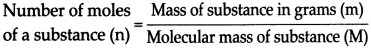Question 3.
What are basic radicals? Give examples.
The radicals which are formed by removal of electrons from the atoms of metals are called as basic radicals, e.g., Na+, Cu2+

Question 4.
What are acidic radicals? Give examples.
The radicals which are formed by adding electrons to the atoms of non-metals are called as acidic radicals, e.g., CT, S2-

State whether the following statement is ‘True’ or ‘False’. Correct the false statement.

(1) Molecular state of oxygen is monoatomic.
(2) The capacity of an element to combine is called its valency.
(4) The magnitude of charge on any radical is its atomic number.
(5) In a chemical reaction, mass of original matter and mass of matter newly formed as a result of chemical change are equal.
(6) The proportion by weight of carbon and oxygen in carbon dioxide is 3 : 5.
(7) Relative mass of hydrogen is 1.
(8) The number of molecules in a given quantity of a substance is determined by its atomic mass.
(9) Avogadro’s number is 6.022 x 1023
(10) Valency of sodium is 2.
(1) False. Molecular state of oxygen is diatomic:
(2) True
(4) False. Magnitude of charge on any radical is its valency.
(5) True
(6) False. The proportion by weight of carbon and oxygen in carbon dioxide is 3 : 8.
(7) True
(8) False. The number of molecules in a given quantity of a substance is determined by its molecular mass.
(9) True
(10) False. Valency of sodium is 1.Name the following:

Question 1.
Scientist who gave Law of Conservation of Matter.
Antoine Lavoisier

Question 2.
Scientist who gave Law of Constant Proportion.
J. L. Proust

Question 3.
What are protons and neutrons present in nucleus together called as?
Nucleons

Question 4.
Unit used to express atomic radius.
Nanometre

Question 5.
The number (p + n) in the atomic nucleus is called as?
Atomic mass number

Question 6.
Name the unit of atomic mass.
Dalton (u)

Question 7.
Write molecular formula of two ionic compounds containing chlorine.
NaCl, MgCl2

Question 8.
Na+, ClQuestion 9.
Give two examples of simple radicals.
Ag+, O2-

Give scientific reasons:

Question 1.
An atom is electrically neutral though it contains charged particles.

• An atom is made up of a nucleus and an extranuclear part. Protons and neutrons are present in the nucleus.
• The nucleus is positively charged. The extranuclear part is made up of negatively charged electrons.
• Protons are positively charged, electrons are negatively charged and neutrons are without any charge.
• The magnitude of their charges is the same when they are equal in number.
• Hence, the negative charge on all the extra, nuclear electrons together balances the positive charge on the
• nucleus.
• Therefore, an atom is electrically neutral though it contains charged particles.

Question 2.
Neon is chemically inert element.

• Atomic number of neon is 10, so its electronic configuration is (2, 8). There are 8 electrons in its 2nd shell, fulfilling its capacity.
• Thus, neon has a complete octet.
• It has a stable orbit therefore, it does not indulge in chemical reactions. Hence, neon is a chemically inert element.

Question 3.
The valency of sodium (Na) is one.

• The electronic configuration of sodium (Na) is (2, 8,1). It has 1 electron in its 3rd orbit.
• It tends to give up this electron so that it is left up with (2, 8), having 8 electrons in the second orbit, with a stable state.
• The loss of one electron leads to the formation of sodium ion (Na+) which is positively charged as it has lost one electron.Question 4.
The valency of chlorine (Cl) is one.

• The electronic configuration of chlorine (Cl) is (2, 8, 7). It has 7 electrons in its 3rd orbit.
• It tends to take one electron from another atom so that it has 8 electrons in the outermost orbit with electronic configuration (2,8,8) with stable state.
• The gaining of one electron leads to formation of chloride ion (Cl) which is negatively charged as it has gained one electron.

Question 5.
The valency of Magnesium (Mg) is two.

• The electronic configuration of Magnesium (Mg) is (2,8,2), it has 2 electrons in its 3rd orbit.
• It tends to give these ‘2’ electrons so that it is left up with (2, 8), having 8 electrons in the second orbit, with a stable state.
• The loss of two electrons leads to the formation of Magnesium ion (Mg2+) which is double positively charged as it has lost two electrons.

Question 6.
Valency is always a whole number.

• The number of electrons that an atom of an element gives away, takes up or shares forming a bond is called the valency of that element.
• These electrons are always in whole numbers and not in fractions.
• Therefore, valency is always a whole number.

Question 7.
Atomic size of potassium is bigger than atomic size of sodium.

• The atomic size of an element depends on the number of electron orbits in the atom of that element.
• The greater the number of orbits, the larger the size.
• Atomic number of potassium (K) is 19. Hence, its electronic configuration is (2, 8, 8,1). While atomic number of sodium (Na) is 11. Hence its electronic configuration is (2, 8,1)
• Number of orbits in potassium atom is 4, while that in sodium atom is 3.
• Hence, atomic size of potassium is bigger than atomic size of sodium.Question 8.
The atomic size of sodium is bigger than atomic size of Magnesium.

• The atomic size of an element depends on the number of electron orbits in the atom of that element.
• If 2 atoms have the same outermost orbit, then the atom having the larger number of electrons in the outermost orbit is smaller than the one having fewer electrons in the same outermost orbit.
• Atomic number of sodium (Na) is 11. Hence, its electronic configuration is (2, 8, 1) while atomic number of magnesum (Mg) is 12 and hence its electronic configuration is (2, 8, 2).
• As compared to sodium atom Magnesum atom has larger number of electrons n its electronic configuration.
• Therefore, atomic size of sodium is bigger than atomic size of Magnesium.

Write the names of the following compounds and deduce their molecular masses:

Atomic masses : H(1), 0(16), N(14), C(12), K(39), S(32), Ca(40), Na(23), C1(35.5), Mg(24), A1(27), P(31)

Question 1.
Molecular mass of K2CO3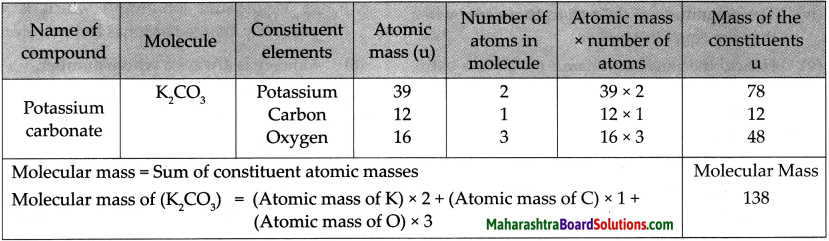Question 2.
Molecular mass of CO2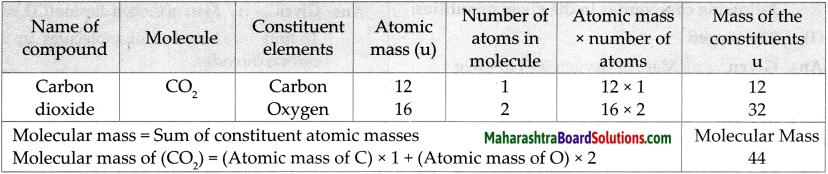Question 3.
Molecular mass of MgCl2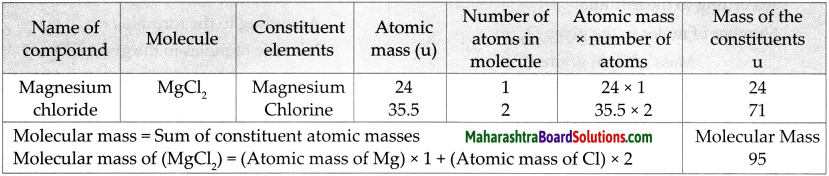Question 4.
Molecular mass of NaOH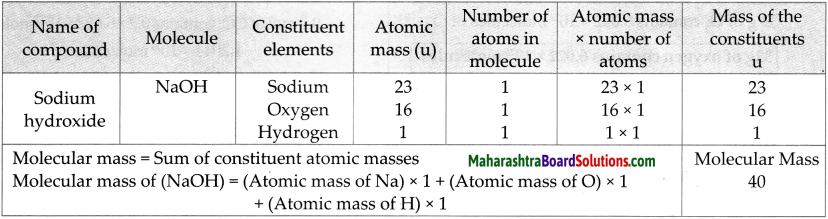Question 5.
Molecular mass of AIPO4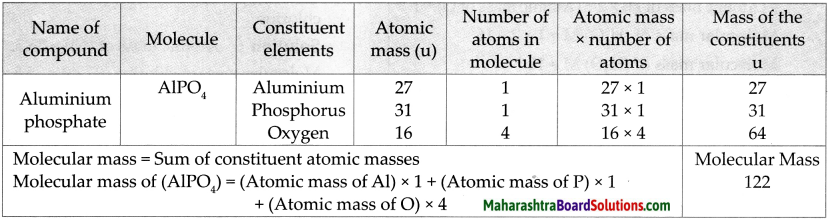Question 6.
Molecular mass of NaHCO3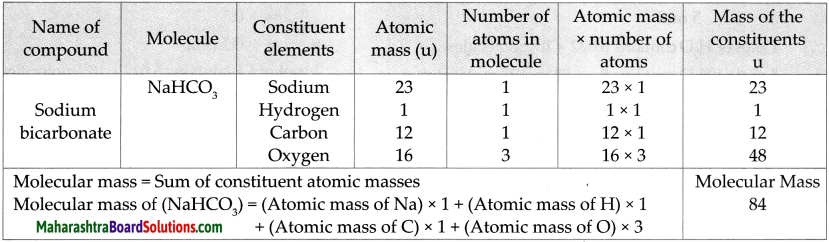Numerical.

Question 1.
Magnesium Oxide:
Given : Number of moles of Magnesium oxide (MgO)n = 0.2 mol
To find : Mass in grams of 0.2 mol of MgO
Solution:
Molecular mass of (MgO)M
= (Atomic mass of Mg) x 1 + (Atomic mass of O) x 1
= 24 x 1 + 16 x 1
= 24 + 16
Molecular mass of (MgO)M = 40
According to the formula Number of moles in the given MgO (n)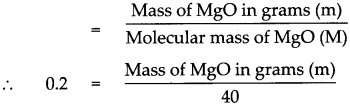Mass of MgO in grams (m) = 0.2 x 40
Mass of MgO in grams (m) = 8 g.
Mass of 0.2 mole of MgO is 8 gQuestion 2.
Calcium Carbonate:
Given : Number of moles of Calcium carbonate (CaCO3) n = 0.2 mol
To find : Mass in grams of 0.2 mol of CaCO3
Solution:
Molecular mass of (CaCO3) M
= (Atomic mass of Ca) x 1 + (Atomic mass of C) x 1 + (Atomic mass of O) x 3
= (40 x l) + (12 x 1) +(16 x 3)
= 40+ 12+ 48
Molecular mass of (CaCO3) M = 100
According to the formula Number of moles in the given CaCO3 (n)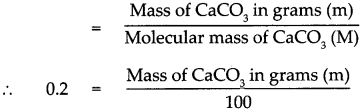∴ Mass of CaCO3 in grams (m) = 0.2 x 100
∴ Mass of CaCO3 in grams (m) = 20 g
Mass of 0.2 mole of CaCO3 is 20 g

State laws/Define the following:

Question 1.
Law of Conservation of Matter.
In a chemical reaction, the total weight of the reactants is same as the total weight of the products formed due to chemical reaction.

Question 2.
Law of Constant Proportion.
The proportion by weight of the constituent elements in the various samples of a compound is fixed.

Question 3.
Molecular Mass:
The molecular mass of a substance is the sum of the atomic masses of all the atoms in a single molecule of that substance.

Question 4.
Mole
A mole is that quantity of a substance whose mass in grams is equal in magnitude to the molecular mass of that substance in Daltons.Question 5.
Valency
The capacity of an element to combine is called its valency.

Question 6.
Electronic definition of Valency
The number of electrons that an atom of an element gives away or takes up while forming an ionic bond is called valency of that element.

Question 7.
The positively or negatively charged ions that take part independently in chemical reactions are called radicals.

Question 8.
Atomic size determination
The size of an atom is determined by its radius. The atomic radius of an isolated atom is the distance between the nucleus of an atom and its outermost orbit.

Question 9.
Atomic mass number
The number of protons and neutrons in the atomic nucleus is called the atomic mass number.

Question 10.
Unified mass
Unified mass is the standard unit of atomic mass that quantifies mass on an atomic or molecular scale. Its symbol is ‘u’.
1 u = 1.66053904 x 10-27 kg.

Question 11.
Molecular mass of a substance
The molecular mass of a substance is the sum of the atomic masses of all the atoms in a single molecule of that substance. Like atomic mass, molecular mass is also expressed in the unit Dalton (u).

Question 1.
What is variable valency?

• Under different conditions, the atoms of some elements give away or take up a different number of electrons.
• In such cases, those elements exhibit more than one valency.
• This property of elements is called variable valency.Complete the following table:

Question 1.
Write down the cations and anions obtained from the compounds in the following chart.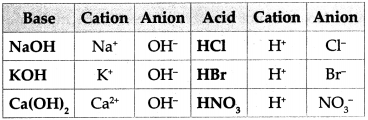Question 1.
Using the chart of ions/radicals and the cross-multiplication method, write the chemical formulae of the following compounds:

(a) Calcium carbonate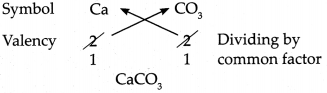∴ Chemical formula of Calcium carbonate is CaCO3

(b) Sodium bicarbonate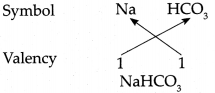∴ Chemical formula of Sodium bicarbonate is NaHCO3

(c) Silver chloride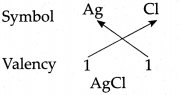∴ Chemical formula of Silver chloride is AgCl(d) Calcium hydroxide Answer: Symbol Ca OH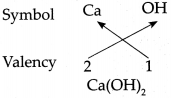∴ Chemical formula of Calcium hydroxide is Ca(OH)2

(e) Magnesium oxide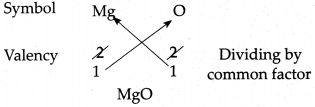∴ Chemical formula of Magnesium oxide is MgO

(f) Ammonium phosphate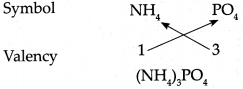∴ Chemical formula of Ammonium phosphate is (NH4)3PO4

(g) Cuprous bromide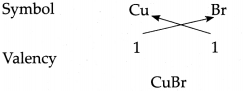∴ Chemical formula of Cuprous bromide is CuBr.

(h) Copper sulphate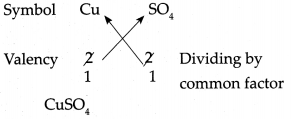∴ Chemical formula of Copper sulphate is CuSO4.

(i) Potassium nitrate